Examples

Chapter 2 Class 9 Polynomials
Serial order wise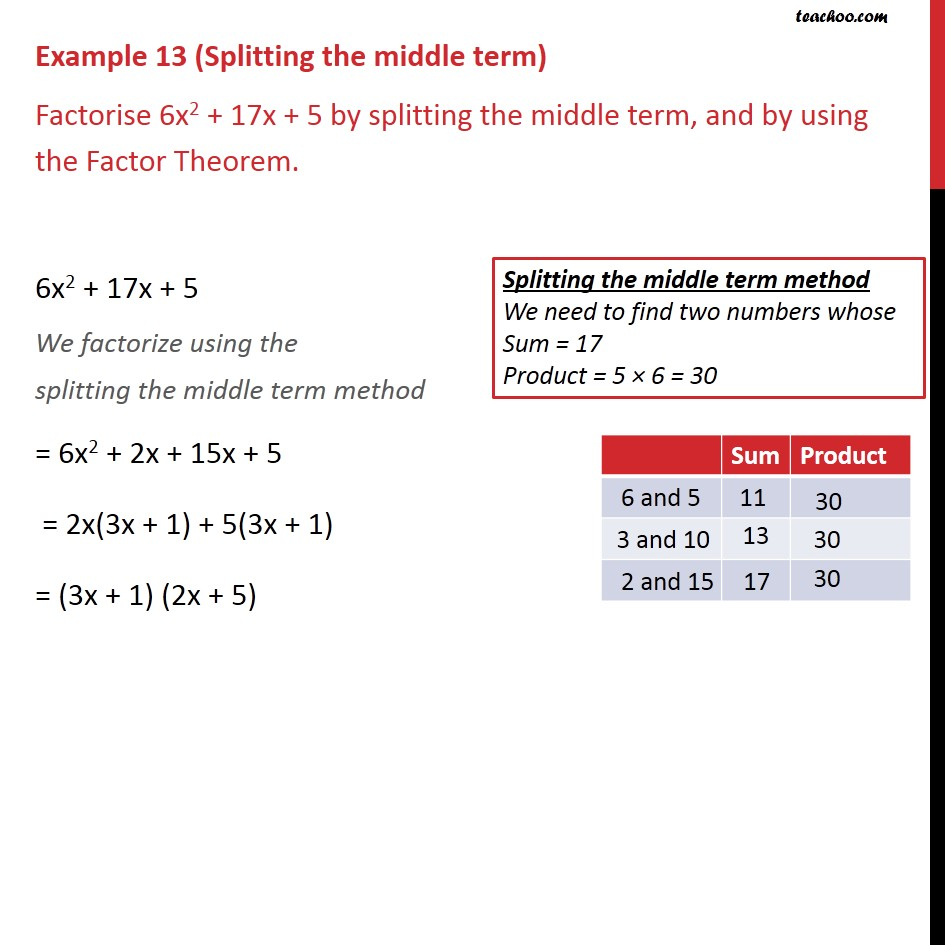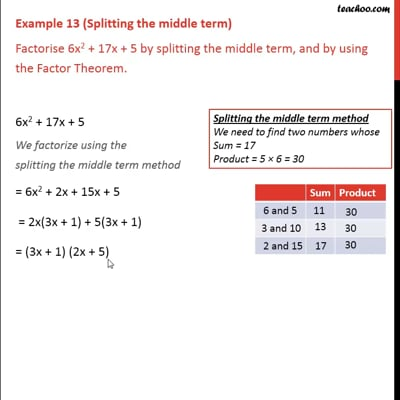This video is only available for Teachoo black users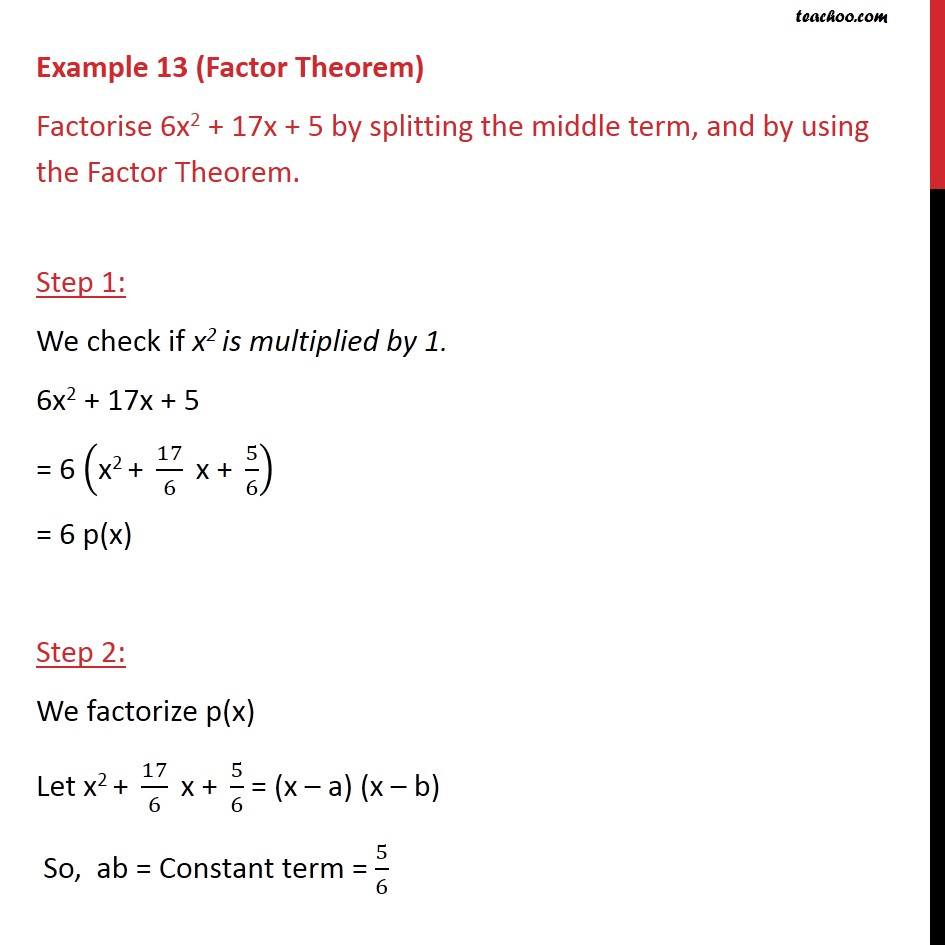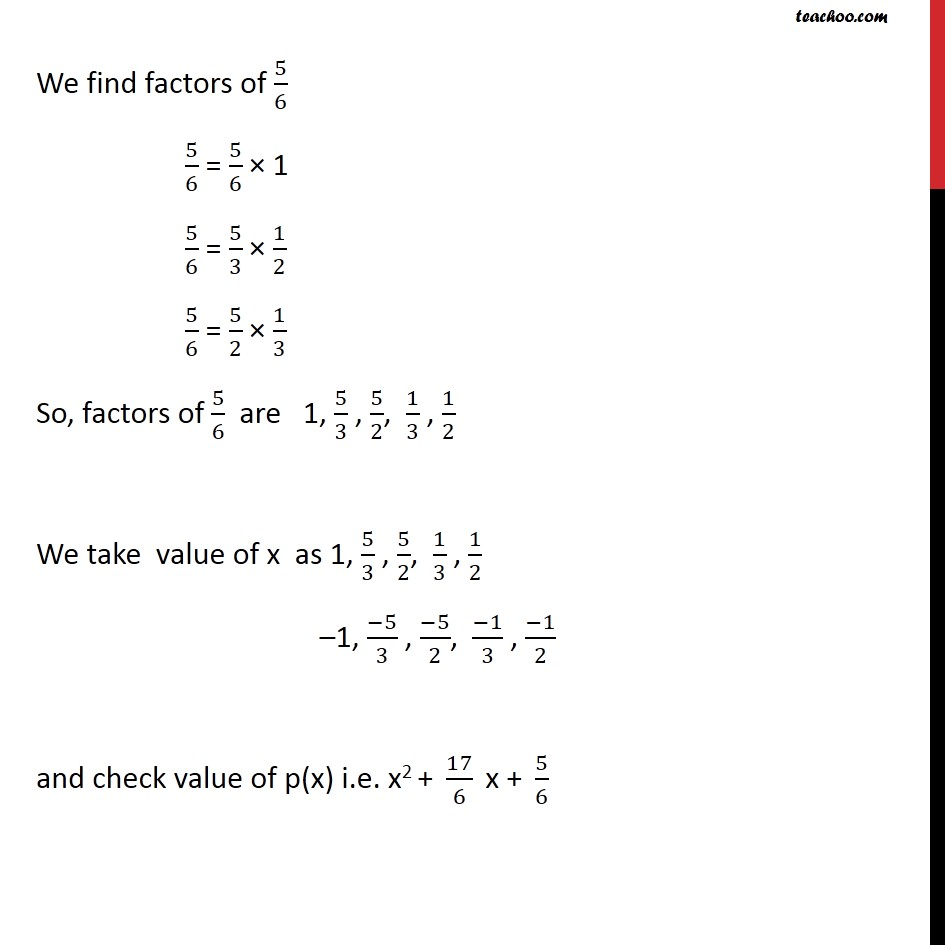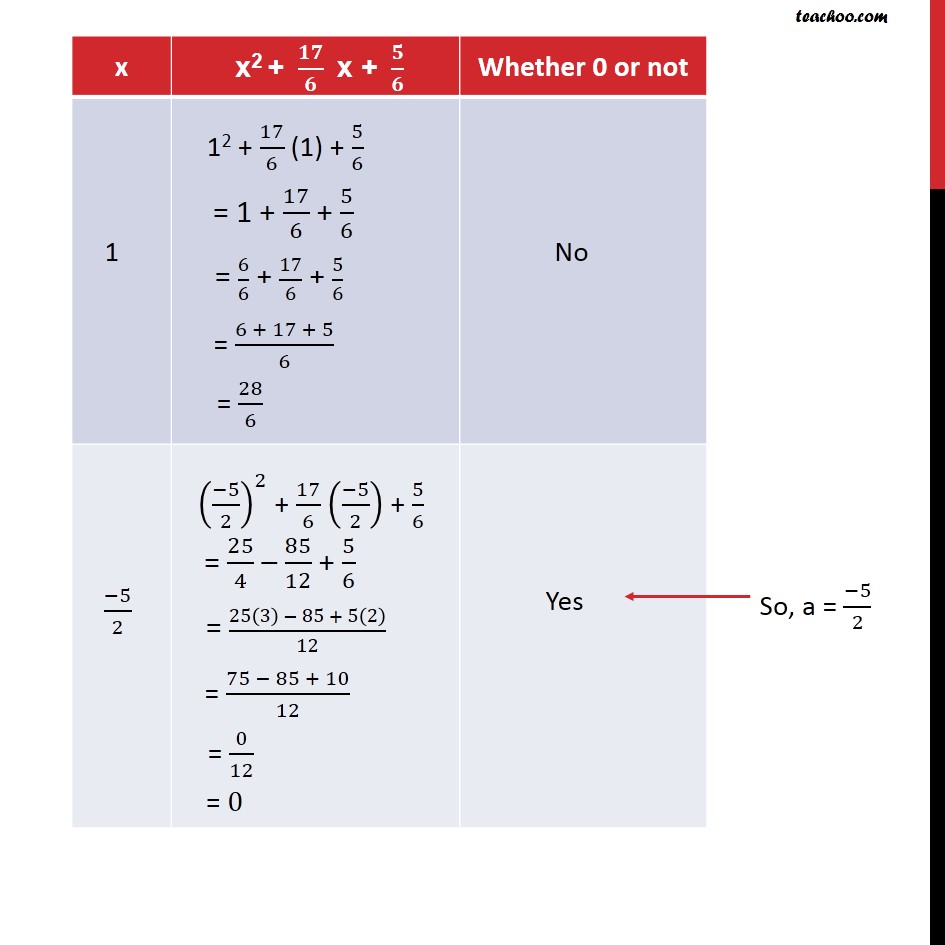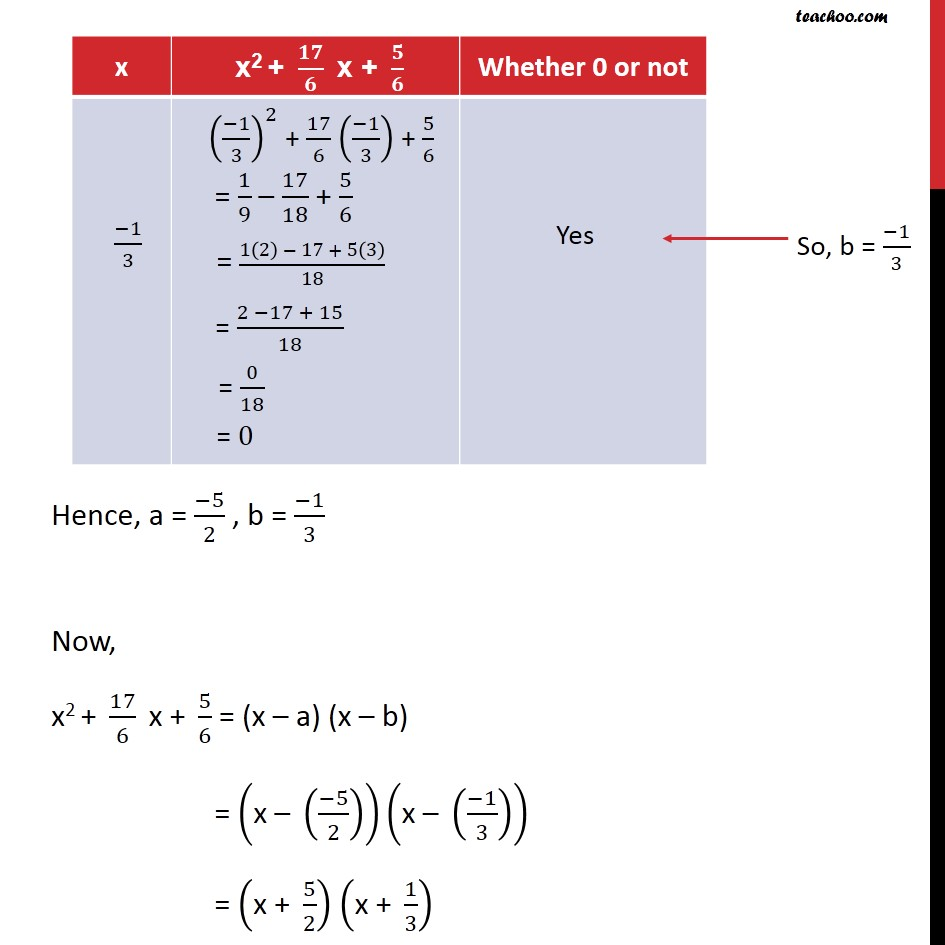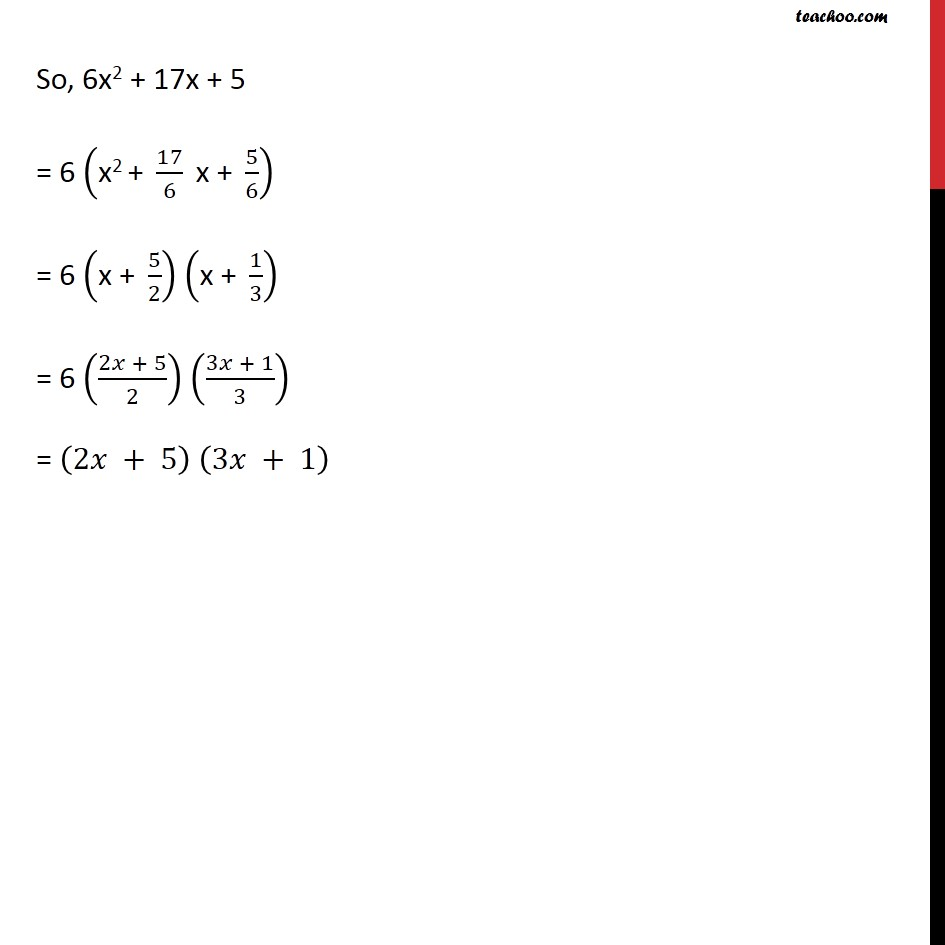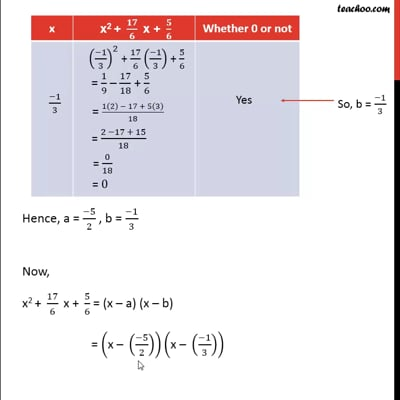This video is only available for Teachoo black users

Maths Crash Course - Live lectures + all videos + Real time Doubt solving!

### Transcript

Example 13 (Splitting the middle term) Factorise 6x2 + 17x + 5 by splitting the middle term, and by using the Factor Theorem. 6x2 + 17x + 5 We factorize using the splitting the middle term method = 6x2 + 2x + 15x + 5 = 2x(3x + 1) + 5(3x + 1) = (3x + 1) (2x + 5) Example 13 (Factor Theorem) Factorise 6x2 + 17x + 5 by splitting the middle term, and by using the Factor Theorem. Step 1: We check if x2 is multiplied by 1. 6x2 + 17x + 5 = 6 ("x2 + " 17/6 " x + " 5/6) = 6 p(x) Step 2: We factorize p(x) Let "x2 + " 17/6 " x + " 5/6 = (x – a) (x – b) So, ab = Constant term = 5/6 We find factors of 5/6 5/6 = 5/6 × 1 5/6 = 5/3 × 1/2 5/6 = 5/2 × 1/3 So, factors of 5/6 are 1, 5/3 , 5/2, 1/3 , 1/2 We take value of x as 1, 5/3 , 5/2, 1/3 , 1/2 –1, (−5)/3 , (−5)/2, (−1)/3 , (−1)/2 and check value of p(x) i.e. "x2 + " 17/6 " x + " 5/6 Hence, a = (−5)/2 , b = (−1)/3 Now, "x2 + " 17/6 " x + " 5/6 = (x – a) (x – b) = ("x – " ((−5)/2))("x – " ((−1)/3)) = ("x + " 5/2) ("x + " 1/3) So, 6x2 + 17x + 5 = 6 ("x2 + " 17/6 " x + " 5/6) = 6 ("x + " 5/2) ("x + " 1/3) = 6 ((2𝑥 + 5)/2) ((3𝑥 + 1)/3) = (2𝑥 + 5) (3𝑥 + 1)